Our prices are denominated in USD.

We ship internationally!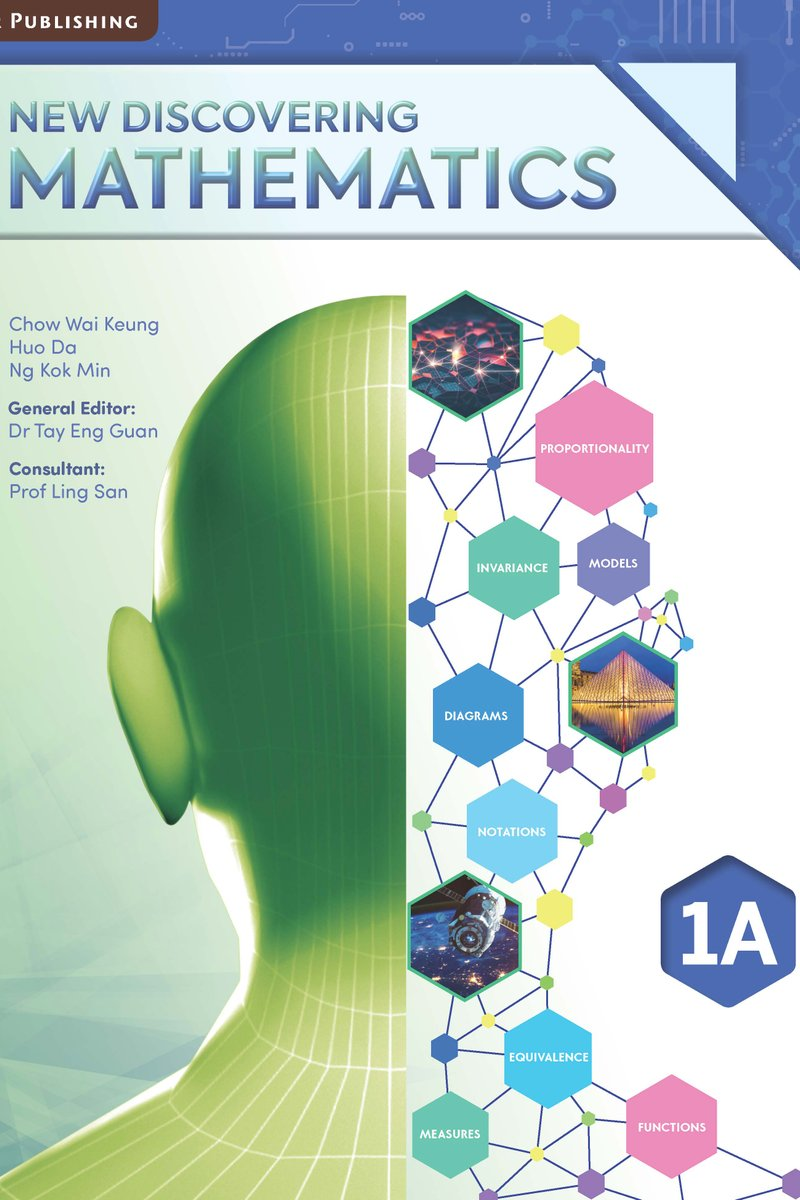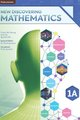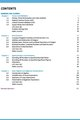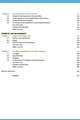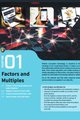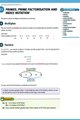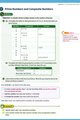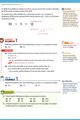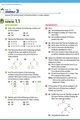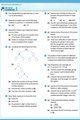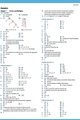# New Discovering Mathematics Textbook 1A

US\$13.50

New Discovering Mathematics is a series of textbooks written with a learner-centric approach that builds upon the knowledge and skills learnt in primary school. It is based on the latest O-level Mathematics syllabus implemented from 2020 by the Ministry of Education of Singapore.

The emphasis of this series is on empowering students to learn mathematics independently and effectively. Depending on the topics covered, different approaches are used to present different concepts to facilitate students' understanding.

New Discovering Mathematics is organised into three content strands: Numbers & Algebra, Geometry & Measurement and Statistics & Probability.

This series includes clear and illustrative examples, class activities and diagrams to help students understand the concepts and apply them.

Key features:

1. Chapter Opener
Introduces a chapter by providing real-world context, focusing on the relevance of mathematics  in our daily lives.

2. Activity Enhances students' learning experiences through exploration and discovery by guiding them through relevant tasks.

3. Worked Example
Illustrates the steps in solving mathematical problems to help students understand a concept or skill. Analysis in difficult problems guides students in unpacking the information and making sense of it.

4. Practice Exercise
Provides exercises with different difficulty levels: Basic, Intermediate and Advanced for further practice.
(Answer key included at the back of book)

5. Let's Sum it Up
Highlight important concepts, formulae, properties and rules for quick revision.

6. Review Exercise
Provides a further exercise to reinforce understanding and consolidate learning for each chapter.

7. Revision Exercise
Tests mastery of what has been learnt with problems that integrate concepts and skills taught across topics.

Includes non-routine problems to develop students' problem-solving skills with prompts to guide students using Polya's 4-step approach.

9. Problems in Real-world Contexts
Gives students opportunities to explore, model and solve problems in real-life situations, allowing them to realise the relevance and utility of mathematics in our daily lives.

10. Professional's Voice
Presents the connections to Big Ideas in a mathematics and provides opportunities to explore how certain concepts relate to and can be applied to other concepts.

11. Core Vocabulary Lists down essential mathematical terms introduced in the chapter.

12. Coding Provides coding opportunities to support development of computational thinking.

13. Discuss Poses questions to extend students' learning and encourage communication of mathematical concepts and ideas.

14. Spotlight Highlights important information to take note of

15. Recall Prompts to recollect concepts and definition learnt previously.

16. Extra Info Introduces scientific, historical or fun mathematical facts related to the topic

For Secondary 1 / Grade 7 / Year 7 / 13 years old

Singapore Curriculum

1. Factors and Multiples
Primes, Factors, Multiples, Roots

2. Real Numbers
Negative numbers, Number Line, Integers, Operations, Rational and Real Numbers

3. Approximation and Estimation
Rounding off to Decimal Places and Significant Figures, Estimation

4. Basic Algebra & Algebraic Manipulation
Use of Letters in Algebra, Algebraic Expressions and Formulae
Like, Unlike Terms, Simplification and Factorisation of Linear Expressions

Revision Exercise 1

5. Simple Equations in One Variable
Simple Linear Equations in One Variable, Brackets, Simple Fractional Equations, Forming Equations

6. Angles and Parallel Lines
Points, Lines, Planes, Angles, Parallel Lines, Perpendicular and Angle Bisectors

7. Triangles and Polygons

Revision Exercise 2

For topics in this book, click here.

Complete series contain:

1. Textbooks (A/B)
2. Workbooks (A/B)
3. Teachers' Guide (outside Singapore only)

The other levels will be progressively released yearly.